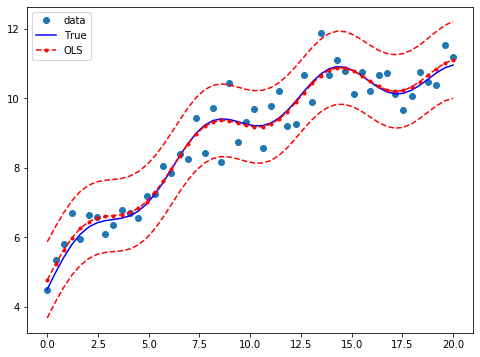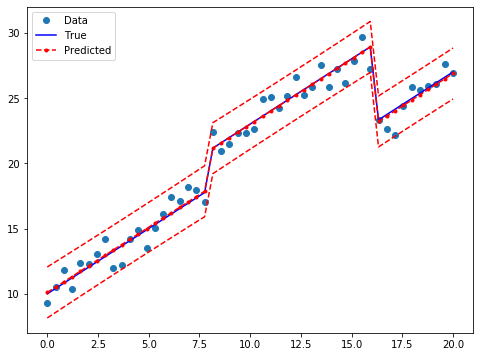Ordinary Least Squares¶

In :
%matplotlib inline
In :
from __future__ import print_function
import numpy as np
import statsmodels.api as sm
import matplotlib.pyplot as plt
from statsmodels.sandbox.regression.predstd import wls_prediction_std

np.random.seed(9876789)

OLS estimation¶

Artificial data:

In :
nsample = 100
x = np.linspace(0, 10, 100)
X = np.column_stack((x, x**2))
beta = np.array([1, 0.1, 10])
e = np.random.normal(size=nsample)

Our model needs an intercept so we add a column of 1s:

In :
y = np.dot(X, beta) + e

Fit and summary:

In :
model = sm.OLS(y, X)
results = model.fit()
print(results.summary())
OLS Regression Results
==============================================================================
Dep. Variable:                      y   R-squared:                       1.000
Method:                 Least Squares   F-statistic:                 4.020e+06
Date:                Sun, 24 Nov 2019   Prob (F-statistic):          2.83e-239
Time:                        07:50:48   Log-Likelihood:                -146.51
No. Observations:                 100   AIC:                             299.0
Df Residuals:                      97   BIC:                             306.8
Df Model:                           2
Covariance Type:            nonrobust
==============================================================================
coef    std err          t      P>|t|      [0.025      0.975]
------------------------------------------------------------------------------
const          1.3423      0.313      4.292      0.000       0.722       1.963
x1            -0.0402      0.145     -0.278      0.781      -0.327       0.247
x2            10.0103      0.014    715.745      0.000       9.982      10.038
==============================================================================
Omnibus:                        2.042   Durbin-Watson:                   2.274
Prob(Omnibus):                  0.360   Jarque-Bera (JB):                1.875
Skew:                           0.234   Prob(JB):                        0.392
Kurtosis:                       2.519   Cond. No.                         144.
==============================================================================

Warnings:
 Standard Errors assume that the covariance matrix of the errors is correctly specified.

Quantities of interest can be extracted directly from the fitted model. Type dir(results) for a full list. Here are some examples:

In :
print('Parameters: ', results.params)
print('R2: ', results.rsquared)
Parameters:  [ 1.34233516 -0.04024948 10.01025357]
R2:  0.9999879365025871

OLS non-linear curve but linear in parameters¶

We simulate artificial data with a non-linear relationship between x and y:

In :
nsample = 50
sig = 0.5
x = np.linspace(0, 20, nsample)
X = np.column_stack((x, np.sin(x), (x-5)**2, np.ones(nsample)))
beta = [0.5, 0.5, -0.02, 5.]

y_true = np.dot(X, beta)
y = y_true + sig * np.random.normal(size=nsample)

Fit and summary:

In :
res = sm.OLS(y, X).fit()
print(res.summary())
OLS Regression Results
==============================================================================
Dep. Variable:                      y   R-squared:                       0.933
Method:                 Least Squares   F-statistic:                     211.8
Date:                Sun, 24 Nov 2019   Prob (F-statistic):           6.30e-27
Time:                        07:50:48   Log-Likelihood:                -34.438
No. Observations:                  50   AIC:                             76.88
Df Residuals:                      46   BIC:                             84.52
Df Model:                           3
Covariance Type:            nonrobust
==============================================================================
coef    std err          t      P>|t|      [0.025      0.975]
------------------------------------------------------------------------------
x1             0.4687      0.026     17.751      0.000       0.416       0.522
x2             0.4836      0.104      4.659      0.000       0.275       0.693
x3            -0.0174      0.002     -7.507      0.000      -0.022      -0.013
const          5.2058      0.171     30.405      0.000       4.861       5.550
==============================================================================
Omnibus:                        0.655   Durbin-Watson:                   2.896
Prob(Omnibus):                  0.721   Jarque-Bera (JB):                0.360
Skew:                           0.207   Prob(JB):                        0.835
Kurtosis:                       3.026   Cond. No.                         221.
==============================================================================

Warnings:
 Standard Errors assume that the covariance matrix of the errors is correctly specified.

Extract other quantities of interest:

In :
print('Parameters: ', res.params)
print('Standard errors: ', res.bse)
print('Predicted values: ', res.predict())
Parameters:  [ 0.46872448  0.48360119 -0.01740479  5.20584496]
Standard errors:  [0.02640602 0.10380518 0.00231847 0.17121765]
Predicted values:  [ 4.77072516  5.22213464  5.63620761  5.98658823  6.25643234  6.44117491
6.54928009  6.60085051  6.62432454  6.6518039   6.71377946  6.83412169
7.02615877  7.29048685  7.61487206  7.97626054  8.34456611  8.68761335
8.97642389  9.18997755  9.31866582  9.36587056  9.34740836  9.28893189
9.22171529  9.17751587  9.1833565   9.25708583  9.40444579  9.61812821
9.87897556 10.15912843 10.42660281 10.65054491 10.8063004  10.87946503
10.86825119 10.78378163 10.64826203 10.49133265 10.34519853 10.23933827
10.19566084 10.22490593 10.32487947 10.48081414 10.66779556 10.85485568
11.01006072 11.10575781]

Draw a plot to compare the true relationship to OLS predictions. Confidence intervals around the predictions are built using the wls_prediction_std command.

In :
prstd, iv_l, iv_u = wls_prediction_std(res)

fig, ax = plt.subplots(figsize=(8,6))

ax.plot(x, y, 'o', label="data")
ax.plot(x, y_true, 'b-', label="True")
ax.plot(x, res.fittedvalues, 'r--.', label="OLS")
ax.plot(x, iv_u, 'r--')
ax.plot(x, iv_l, 'r--')
ax.legend(loc='best');OLS with dummy variables¶

We generate some artificial data. There are 3 groups which will be modelled using dummy variables. Group 0 is the omitted/benchmark category.

In :
nsample = 50
groups = np.zeros(nsample, int)
groups[20:40] = 1
groups[40:] = 2
#dummy = (groups[:,None] == np.unique(groups)).astype(float)

dummy = sm.categorical(groups, drop=True)
x = np.linspace(0, 20, nsample)
# drop reference category
X = np.column_stack((x, dummy[:,1:]))

beta = [1., 3, -3, 10]
y_true = np.dot(X, beta)
e = np.random.normal(size=nsample)
y = y_true + e

Inspect the data:

In :
print(X[:5,:])
print(y[:5])
print(groups)
print(dummy[:5,:])
[[0.         0.         0.         1.        ]
[0.40816327 0.         0.         1.        ]
[0.81632653 0.         0.         1.        ]
[1.2244898  0.         0.         1.        ]
[1.63265306 0.         0.         1.        ]]
[ 9.28223335 10.50481865 11.84389206 10.38508408 12.37941998]
[0 0 0 0 0 0 0 0 0 0 0 0 0 0 0 0 0 0 0 0 1 1 1 1 1 1 1 1 1 1 1 1 1 1 1 1 1
1 1 1 2 2 2 2 2 2 2 2 2 2]
[[1. 0. 0.]
[1. 0. 0.]
[1. 0. 0.]
[1. 0. 0.]
[1. 0. 0.]]

Fit and summary:

In :
res2 = sm.OLS(y, X).fit()
print(res2.summary())
OLS Regression Results
==============================================================================
Dep. Variable:                      y   R-squared:                       0.978
Method:                 Least Squares   F-statistic:                     671.7
Date:                Sun, 24 Nov 2019   Prob (F-statistic):           5.69e-38
Time:                        07:50:48   Log-Likelihood:                -64.643
No. Observations:                  50   AIC:                             137.3
Df Residuals:                      46   BIC:                             144.9
Df Model:                           3
Covariance Type:            nonrobust
==============================================================================
coef    std err          t      P>|t|      [0.025      0.975]
------------------------------------------------------------------------------
x1             0.9999      0.060     16.689      0.000       0.879       1.121
x2             2.8909      0.569      5.081      0.000       1.746       4.036
x3            -3.2232      0.927     -3.477      0.001      -5.089      -1.357
const         10.1031      0.310     32.573      0.000       9.479      10.727
==============================================================================
Omnibus:                        2.831   Durbin-Watson:                   1.998
Prob(Omnibus):                  0.243   Jarque-Bera (JB):                1.927
Skew:                          -0.279   Prob(JB):                        0.382
Kurtosis:                       2.217   Cond. No.                         96.3
==============================================================================

Warnings:
 Standard Errors assume that the covariance matrix of the errors is correctly specified.

Draw a plot to compare the true relationship to OLS predictions:

In :
prstd, iv_l, iv_u = wls_prediction_std(res2)

fig, ax = plt.subplots(figsize=(8,6))

ax.plot(x, y, 'o', label="Data")
ax.plot(x, y_true, 'b-', label="True")
ax.plot(x, res2.fittedvalues, 'r--.', label="Predicted")
ax.plot(x, iv_u, 'r--')
ax.plot(x, iv_l, 'r--')
legend = ax.legend(loc="best")Joint hypothesis test¶

F test¶

We want to test the hypothesis that both coefficients on the dummy variables are equal to zero, that is, $R \times \beta = 0$. An F test leads us to strongly reject the null hypothesis of identical constant in the 3 groups:

In :
R = [[0, 1, 0, 0], [0, 0, 1, 0]]
print(np.array(R))
print(res2.f_test(R))
[[0 1 0 0]
[0 0 1 0]]
<F test: F=array([[145.49268198]]), p=1.2834419617291377e-20, df_denom=46, df_num=2>

You can also use formula-like syntax to test hypotheses

In :
print(res2.f_test("x2 = x3 = 0"))
<F test: F=array([[145.49268198]]), p=1.2834419617291078e-20, df_denom=46, df_num=2>

Small group effects¶

If we generate artificial data with smaller group effects, the T test can no longer reject the Null hypothesis:

In :
beta = [1., 0.3, -0.0, 10]
y_true = np.dot(X, beta)
y = y_true + np.random.normal(size=nsample)

res3 = sm.OLS(y, X).fit()
In :
print(res3.f_test(R))
<F test: F=array([[1.22491119]]), p=0.3031864410632063, df_denom=46, df_num=2>
In :
print(res3.f_test("x2 = x3 = 0"))
<F test: F=array([[1.22491119]]), p=0.3031864410632063, df_denom=46, df_num=2>

Multicollinearity¶

The Longley dataset is well known to have high multicollinearity. That is, the exogenous predictors are highly correlated. This is problematic because it can affect the stability of our coefficient estimates as we make minor changes to model specification.

In :
/home/travis/miniconda/envs/statsmodels-test/lib/python3.7/site-packages/numpy/core/fromnumeric.py:2389: FutureWarning: Method .ptp is deprecated and will be removed in a future version. Use numpy.ptp instead.
return ptp(axis=axis, out=out, **kwargs)

Fit and summary:

In :
ols_model = sm.OLS(y, X)
ols_results = ols_model.fit()
print(ols_results.summary())
OLS Regression Results
==============================================================================
Dep. Variable:                 TOTEMP   R-squared:                       0.995
Method:                 Least Squares   F-statistic:                     330.3
Date:                Sun, 24 Nov 2019   Prob (F-statistic):           4.98e-10
Time:                        07:50:49   Log-Likelihood:                -109.62
No. Observations:                  16   AIC:                             233.2
Df Residuals:                       9   BIC:                             238.6
Df Model:                           6
Covariance Type:            nonrobust
==============================================================================
coef    std err          t      P>|t|      [0.025      0.975]
------------------------------------------------------------------------------
const      -3.482e+06    8.9e+05     -3.911      0.004    -5.5e+06   -1.47e+06
GNPDEFL       15.0619     84.915      0.177      0.863    -177.029     207.153
GNP           -0.0358      0.033     -1.070      0.313      -0.112       0.040
UNEMP         -2.0202      0.488     -4.136      0.003      -3.125      -0.915
ARMED         -1.0332      0.214     -4.822      0.001      -1.518      -0.549
POP           -0.0511      0.226     -0.226      0.826      -0.563       0.460
YEAR        1829.1515    455.478      4.016      0.003     798.788    2859.515
==============================================================================
Omnibus:                        0.749   Durbin-Watson:                   2.559
Prob(Omnibus):                  0.688   Jarque-Bera (JB):                0.684
Skew:                           0.420   Prob(JB):                        0.710
Kurtosis:                       2.434   Cond. No.                     4.86e+09
==============================================================================

Warnings:
 Standard Errors assume that the covariance matrix of the errors is correctly specified.
 The condition number is large, 4.86e+09. This might indicate that there are
strong multicollinearity or other numerical problems.
/home/travis/miniconda/envs/statsmodels-test/lib/python3.7/site-packages/scipy/stats/stats.py:1416: UserWarning: kurtosistest only valid for n>=20 ... continuing anyway, n=16
"anyway, n=%i" % int(n))

Condition number¶

One way to assess multicollinearity is to compute the condition number. Values over 20 are worrisome (see Greene 4.9). The first step is to normalize the independent variables to have unit length:

In :
norm_x = X.values
for i, name in enumerate(X):
if name == "const":
continue
norm_x[:,i] = X[name]/np.linalg.norm(X[name])
norm_xtx = np.dot(norm_x.T,norm_x)

Then, we take the square root of the ratio of the biggest to the smallest eigen values.

In :
eigs = np.linalg.eigvals(norm_xtx)
condition_number = np.sqrt(eigs.max() / eigs.min())
print(condition_number)
56240.8709117813

Dropping an observation¶

Greene also points out that dropping a single observation can have a dramatic effect on the coefficient estimates:

In :
ols_results2 = sm.OLS(y.iloc[:14], X.iloc[:14]).fit()
print("Percentage change %4.2f%%\n"*7 % tuple([i for i in (ols_results2.params - ols_results.params)/ols_results.params*100]))
Percentage change 4.55%
Percentage change -2228.01%
Percentage change 154304695.31%
Percentage change 1366329.02%
Percentage change 1112549.36%
Percentage change 92708715.91%
Percentage change 817944.26%

We can also look at formal statistics for this such as the DFBETAS -- a standardized measure of how much each coefficient changes when that observation is left out.

In :
infl = ols_results.get_influence()

In general we may consider DBETAS in absolute value greater than $2/\sqrt{N}$ to be influential observations

In :
2./len(X)**.5
Out:
0.5
In :
print(infl.summary_frame().filter(regex="dfb"))
dfb_const  dfb_GNPDEFL       dfb_GNP     dfb_UNEMP     dfb_ARMED  \
0   -0.016406  -169.822675  1.673981e+06  54490.318088  51447.824036
1   -0.020608  -187.251727  1.829990e+06  54495.312977  52659.808664
2   -0.008382   -65.417834  1.587601e+06  52002.330476  49078.352378
3    0.018093   288.503914  1.155359e+06  56211.331922  60350.723082
4    1.871260  -171.109595  4.498197e+06  82532.785818  71034.429294
5   -0.321373  -104.123822  1.398891e+06  52559.760056  47486.527649
6    0.315945  -169.413317  2.364827e+06  59754.651394  50371.817827
7    0.015816   -69.343793  1.641243e+06  51849.056936  48628.749338
8   -0.004019   -86.903523  1.649443e+06  52023.265116  49114.178265
9   -1.018242  -201.315802  1.371257e+06  56432.027292  53997.742487
10   0.030947   -78.359439  1.658753e+06  52254.848135  49341.055289
11   0.005987  -100.926843  1.662425e+06  51744.606934  48968.560299
12  -0.135883   -32.093127  1.245487e+06  50203.467593  51148.376274
13   0.032736   -78.513866  1.648417e+06  52509.194459  50212.844641
14   0.305868   -16.833121  1.829996e+06  60975.868083  58263.878679
15  -0.538323   102.027105  1.344844e+06  54721.897640  49660.474568

dfb_POP      dfb_YEAR
0   207954.113590 -31969.158503
1    25343.938291 -29760.155888
2   107465.770565 -29593.195253
3   456190.215133 -36213.129569
4  -389122.401699 -49905.782854
5   144354.586054 -28985.057609
6  -107413.074918 -32984.462465
7    92843.959345 -29724.975873
8    83931.635336 -29563.619222
9    18392.575057 -29203.217108
10   93617.648517 -29846.022426
11   95414.217290 -29690.904188
12  258559.048569 -29296.334617
13  104434.061226 -30025.564763
14  275103.677859 -36060.612522
15 -110176.960671 -28053.834556
/home/travis/build/statsmodels/statsmodels/statsmodels/stats/outliers_influence.py:695: RuntimeWarning: invalid value encountered in sqrt
return self.resid / sigma / np.sqrt(1 - hii)
/home/travis/miniconda/envs/statsmodels-test/lib/python3.7/site-packages/scipy/stats/_distn_infrastructure.py:877: RuntimeWarning: invalid value encountered in greater
return (self.a < x) & (x < self.b)
/home/travis/miniconda/envs/statsmodels-test/lib/python3.7/site-packages/scipy/stats/_distn_infrastructure.py:877: RuntimeWarning: invalid value encountered in less
return (self.a < x) & (x < self.b)
/home/travis/miniconda/envs/statsmodels-test/lib/python3.7/site-packages/scipy/stats/_distn_infrastructure.py:1831: RuntimeWarning: invalid value encountered in less_equal
cond2 = cond0 & (x <= self.a)
/home/travis/build/statsmodels/statsmodels/statsmodels/stats/outliers_influence.py:729: RuntimeWarning: invalid value encountered in sqrt
dffits_ = self.resid_studentized_internal * np.sqrt(hii / (1 - hii))
/home/travis/build/statsmodels/statsmodels/statsmodels/stats/outliers_influence.py:758: RuntimeWarning: invalid value encountered in sqrt
dffits_ = self.resid_studentized_external * np.sqrt(hii / (1 - hii))